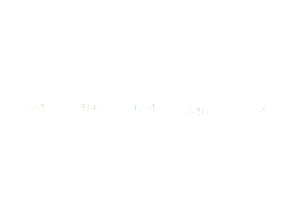Home > Math Shortcuts > Brain Trigger #27 – Sample Number puzzle question

# Brain Trigger #27 – Sample Number puzzle question

Now here we will discuss few question answers on Number puzzle which are very common in competitive exams. Without these type of Number puzzle question your exam preparation will not complete. Try to Solve these questions with your math skills. You can use shortcut methods to solve these Number puzzle questions.

We try to bring together all types of shortcut methods on Number puzzle for every topic here in this website. Now just apply shortcut tricks of Number puzzle to solve these Number puzzle problems. Without applying any shortcut tricks, you can also solve these questions.

Here we will discuss a question on Number puzzle. What you need to do is to, read the question carefully and then try yourself to solve the question. We will provide the answer of this question below. If you try this question and get a answer then check your answer with our answer. If you don’t know how to do it then check the solution below.

Each page is having a question on Number puzzle and also contain its solution with detail explanation. Next/Previous link will help you to navigate through other questions.

Let’s begin question with answer session. Today’s question is:

What should be the number replaces in the question mark ?To know the solution of this problem please scroll down below. You can take as much time as you need to solve the problem. But try to solve this as quicker as you can.

(Scroll down to get the answer)

(Scroll down to get the answer)

(Scroll down to get the answer)

(Scroll down to get the answer)

Now here we will discuss the answer of above question. If you already done this by yourself then please check your answer with us. If you got an correct answer then very Well Done, but don’t worry if you are not able to answer the question correctly. Here is the solution of the problem with explanation.

You can solve in this way…..

2 is increased first digit of left most three digit number to the second three digit number
and middle number decrease by 1 and right digit is alternate with 4 and 6

274 466 654 846 1034

 << PREVIOUS QUESTION NEXT QUESTION >>

Questions on some diferrent topics is also provided here. Click on Next/Previous link, it will take you to different topics.